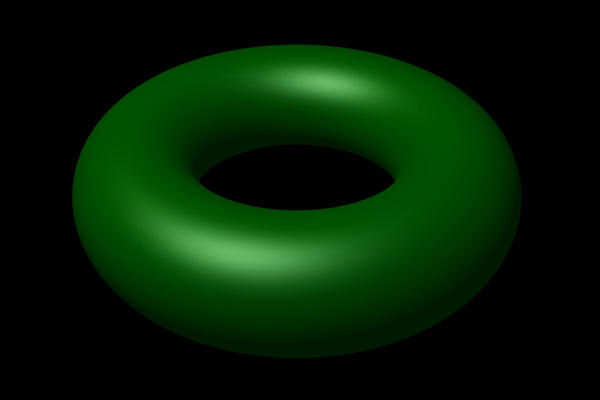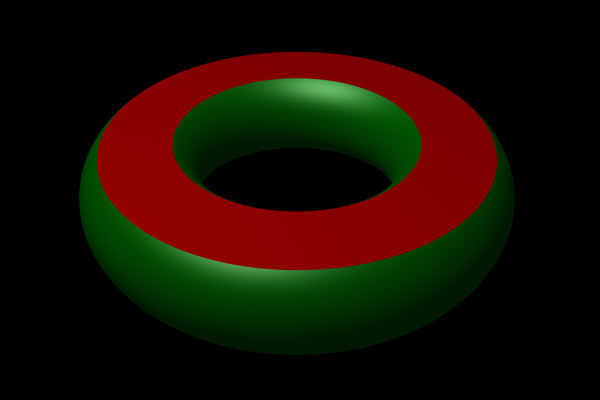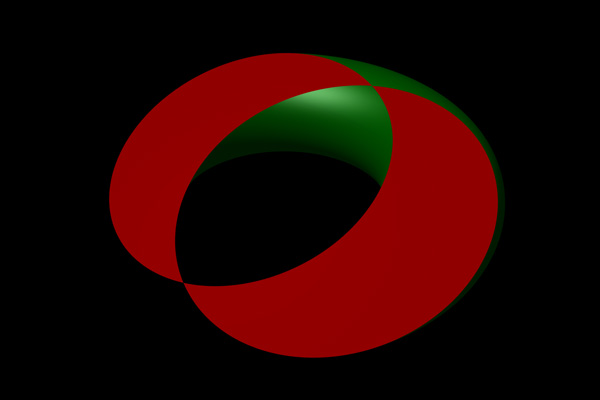# Slicing a Torus

or

### How many ways can a torus be cut (with a single plane) so that the resulting cross sections are perfect circles?

Written by Paul Bourke
November 2003

Update, June 2008: Appeared in The Scottish Mathematical Council Journal #37, pp 62.

Answer: There are three ways to cut a torus (any torus) with a single plane in such a way that the resulting cross section consist only of circles. The first two solutions are fairly obvious, the third solution can be surprising. An example torus is given below followed by images illustrating the three cuts. The images here were all created using the following PovRay source code: torus.ini and torus.pov.Torus, r1 = major radius = 2, r2 = minor radius = 0.75

1. "Horizontal" slice resulting in two concentric circles.The radii of the two circles is given by

r1 + sqrt(r22 - h2)
and
r1 - sqrt(r22 - h2)

where h is the distance of the cutting plane above the plane of the torus. Note that when the cutting plane is at a distance equal to the minor radius r2 then there is only one solution, at greater distances there are no solutions (the plane doesn't cut the torus).

2. "Vertical" slice resulting in two non-intersecting circles.